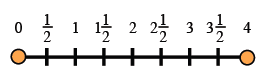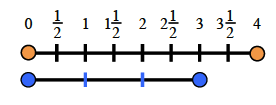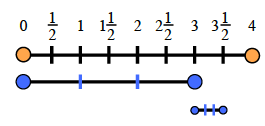### Home > CC1MN > Chapter 5 Unit 5B > Lesson CC1: 5.3.2 > Problem5-81

5-81.

Additional Challenge: Jill lives $3\frac { 1 } { 2 }$ miles from school. One morning, her friend was giving her a ride. When they were $\frac { 2 } { 3 }$ of the way to school, their car broke down and they had to walk the rest of the way. Draw a picture to help you figure out how far they walked.

$\text{If Jill and her friend were able to drive }\frac{2}{3}\text{ of the way to school, then they had to walk }\frac{1}{3}\text{ of the way.}$

$\text{The number line below is numbered 0 through 4. In order to find the total value of }\frac{1}{3}\text{ of } 3\frac{1}{2},$

$\text{First, find } \frac{1}{3}\text{ of } 3.$

$\text{Now find } \frac{1}{3}\text{ of } \frac{1}{2}.$

Were you able to find the distance Jill walked?
Remember to draw a picture of your own!